# RD Sharma Solutions Class 7 Chapter 16 Congruence

Read RD Sharma Solutions Class 7 Chapter 16 Congruence below, students should study RD Sharma class 7 Mathematics available on Studiestoday.com with solved questions and answers. These chapter wise answers for class 7 Mathematics have been prepared by teacher of Grade 7. These RD Sharma class 7 Solutions have been designed as per the latest NCERT syllabus for class 7 and if practiced thoroughly can help you to score good marks in standard 7 Mathematics class tests and examinations

Exercise 16.1

Question :1. Explain the concept of congruence of figures with the help of certain examples.

Solution  1:

Congruent objects or figures are identical replicas of one another, or mirror images of one another. Congruence is the property of two things being congruent.

Take a look at Balls 1 and 2. These two balls are the same size.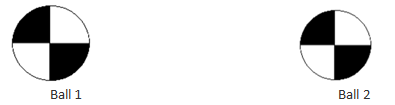Take a look at the two stars below. The scale, colour, and shape of Star A and Star B are identical. There are stars that are in sync.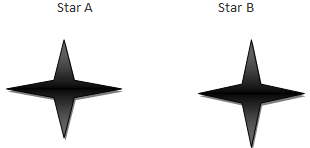Question :2. Fill in the blanks:

(i) Two-line segments are congruent if ___________.

(ii) Two angles are congruent if ___________.

(iii) Two squares are congruent if ___________.

(iv) Two rectangles are congruent if ___________.

(v) Two circles are congruent if ___________.

Solution 2:

(i)Two-line segments are congruent if they are of equal lengths.

(ii)Two angles are congruent if their measures are the same or equal.

(iii)Two squares are congruent if their sides are equal or they have the same side length.

(iv)Two rectangles are congruent if their dimensions are same that is lengths are equal and their breadths are also equal.

(v)Two circles are congruent if they have same radii.

Question :3. In Fig. 6, ∠POQ ≅∠ROS, can we say that ∠POR ≅∠QOS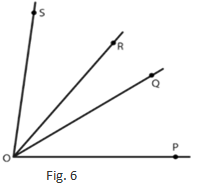Solution 3:

According to question: ∠POQ ≅∠ROS

Also ∠ROQ ≅∠ROQ

Thus, adding ∠ROQ to both sides of ∠POQ ≅∠ROS,

∠POQ + ∠ROQ ≅∠ROQ + ∠ROS

Thus, ∠POR ≅∠QOS

Question :4. In fig. 7, a = b = c, name the angle which is congruent to ∠AOCSolution 4:

According to the figure,

∠ AOB = ∠ BOC = ∠ COD

Thus, ∠ AOB = ∠COD

Also, ∠ AOB + ∠ BOC = ∠ BOC + ∠ COD

∠ AOC = ∠ BOD

Hence, ∠ BOD ≅∠ AOC

Question :5. Is it correct to say that any two right angles are congruent? Give reasons to justify your answer.

Solution  5:

Two right angles are congruent to each other since they both measure 90o.

We know that two angles are congruent if they have the same measure.

Question :6. In fig. 8, ∠AOC ≅∠PYR and ∠BOC ≅∠QYR. Name the angle which is congruent to ∠AOB.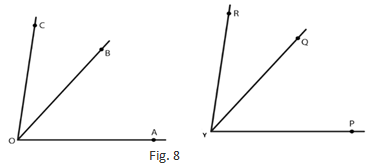Solution 6:

In the given information

∠AOC ≅∠PYR

Also, ∠BOC ≅∠QYR

∠AOC = ∠AOB + ∠BOC and ∠PYR =∠PYQ +∠QYR

On substitute the value of ∠AOC and ∠PYR in ∠AOC ≅∠PYR

∠AOB + ∠BOC ≅∠PYQ + ∠QYR

∠AOB ≅∠PYQ (∠BOC ≅∠QYR)

So, ∠AOB ≅∠PYQ

Question :7. Which of the following statements are true and which are false;

(i) All squares are congruent.

(ii) If two squares have equal areas, they are congruent.

(iii) If two rectangles have equal areas, they are congruent.

(iv) If two triangles have equal areas, they are congruent.

Solution 7:

(i) All squares are congruent.

Clarification:

A square's sides are all the same length. Different squares, on the other hand, may have different lengths of sides. As a result, no squares are congruent.  False

(ii) If two squares have equal areas, they are congruent.  True

(iii) If two rectangles have equal areas, they are congruent.

Clarification:

Area of a rectangle = length x breadth

The region of two rectangles may be the same. However, the lengths of their sides will differ, making them incompatible. False

(iv) If two triangles have equal areas, they are congruent.

Clarification:

Area of a triangle = 1/2 x base x height.

Two triangles may have the same area, but their side lengths may differ, preventing them from being congruent. False

Exercise 16.2

Question :1. In the following pairs of triangles (Fig. 12 to 15), the lengths of the sides are indicated along sides. By applying SSS condition, determine which are congruent. State the result in symbolic form.

Solution 1:(i) In Δ ABC and Δ DEF

AB = DE (each 4.5 cm)

BC = EF (each 6 cm)

AC = DF (each 4 cm)

The SSS criteria states that two triangles are congruent if their three sides are identical.

Thus, According to SSS standard of congruence, ΔABC ≅ ΔDEF

(ii) In Δ ACB and Δ ADB

AC = AD (each 5.5 cm)

BC = BD (each 5 cm)

AB = AB (each 6 cm)

The SSS criteria states that two triangles are congruent if their three sides are identical.

Thus, by SSS standard of congruence, ΔACB ≅ ΔADB

(iii) In Δ ABD and Δ FEC,

AB = FE (each 5 cm)

AD = FC (each 10.5 cm)

BD = CE (each 7 cm)

The SSS criteria states that two triangles are congruent if their three sides are identical.

Thus, According to SSS standard of congruence, ΔABD ≅ ΔFEC

(iv) In Δ ABO and Δ DOC,

AB = DC (each 4 cm)

AO = OC (each 2 cm)

BO = OD (each 3.5 cm)

The SSS criteria states that two triangles are congruent if their three sides are identical.

Thus, According to SSS standard of congruence, ΔABO ≅ ΔODC

Question :2. In fig.16, AD = DC and AB = BC

(i) Is ΔABD ≅ ΔCBD?

(ii) State the three parts of matching pairs you have used to answer (i).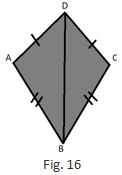Solution 2:

(i) Yes, ΔABD ≅ΔCBD by the SSS criterion.

The SSS criteria states that two triangles are congruent if their three sides are identical.

So, ΔABD ≅ΔCBD

(ii) We have used the three conditions in the SSS criterion as follows:

AD = DC;              AB = BC; and                      DB = BD

Question :3. In Fig. 17, AB = DC and BC = AD.

(i) Is ΔABC ≅ ΔCDA?

(ii) What congruence condition have you used?

(iii) You have used some fact, not given in the question, what is that?Solution 3:

(i) According to the figure AB = DC

And AC = CA

The SSS criteria states that two triangles are congruent if their three sides are identical.

Thus, by SSS standard of congruence ΔABC ≅ ΔCDA

(ii) By using Side Side Side standard of congruence condition with one side common in both the triangles.

(iii)Yes, we have used the fact that AC = CA.

Question :4. In ΔPQR ≅ ΔEFD,

(i) Which side of ΔPQR equals ED?

(ii) Which angle of ΔPQR equals angle E?

Solution 4: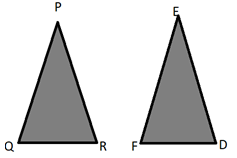(i) PR = ED

Since congruent triangles' corresponding sides are equal.

(ii) ∠QPR = ∠FED

Since congruent triangles' corresponding angles are equal.

Question :5. Triangles ABC and PQR are both isosceles with AB = AC and PQ = PR respectively. If also, AB = PQ and BC = QR, are the two triangles congruent? Which condition do you use?

It ∠B = 50°, what is the measure of ∠R?

Solution 5:

According to the question AB = AC in isosceles ΔABC

And PQ = PR in isosceles ΔPQR.

Also, AB = PQ and QR = BC.

Thus, AC = PR (AB = AC, PQ = PR and AB = PQ)

So, ΔABC ≅ ΔPQR

∠ABC = ∠PQR                    [Since triangles are congruent]

Though, ΔPQR is isosceles.

Thus, ∠PRQ = ∠PQR = ∠ABC = 50o

Question :6. ABC and DBC are both isosceles triangles on a common base BC such that A and D lie on the same side of BC. Are triangles ADB and ADC congruent? Which condition do you use? If ∠BAC = 40° and ∠BDC = 100°, then find ∠ADB.

Solution 6:

According to the question ABC and DBC are both isosceles triangles on a common base BC

∠ABC + ∠BCA + ∠BAC = 180o                      [Angle sum property]

Then ΔABC is an isosceles triangle,

∠ABC = ∠BCA

∠ABC +∠ABC + 40= 180o

2 ∠ABC = 180o– 40o = 140o

∠ABC = 140/2  = 70o

∠DBC + ∠ BCD + ∠ BDC = 180o                    [Angle sum property]

Then ΔDBC is an isosceles triangle, ∠ DBC = ∠BCD

∠DBC + ∠DBC + 100= 180o

2 ∠DBC = 180°– 100o = 80o

∠DBC = 80/2 = 40o

30o + 20o + ∠ADB = 180o                                 [∠ADB = ∠ABC – ∠DBC]

Question :7. Δ ABC and ΔABD are on a common base AB, and AC = BD and BC = AD as shown in Fig. 18. Which of the following statements is true?

(i) ΔABC ≅ ΔABD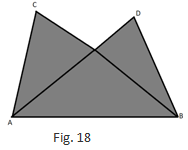Solution 7:

In ΔABC and ΔBAD we have,

AC = BD;               [According to question]

BC = AD;               [According to question]

AB = BA                [CPCT]

Thus, by SSS standard of congruency, ΔABC ≅ ΔBAD

Thus option (iii) is true.

Question :8. In Fig. 19, ΔABC is isosceles with AB = AC, D is the mid-point of base BC.

(ii) State the three pairs of matching parts you use to arrive at your answer.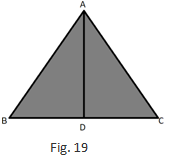Solution 8:

(i) AB = AC           [According to the question]

then D is the midpoint of BC, BD = DC

Thus, by SSS standard of congruency condition,

(ii) AB, AC; BD, DC and AD, DA

Question :9. In fig. 20, ΔABC is isosceles with AB = AC. State if ΔABC ≅ ΔACB. If yes, state three relations that you use to arrive at your answer.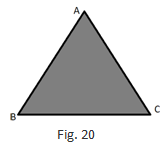Solution 9:

According to the question ΔABC is isosceles with AB = AC

SSS standard is two triangles are congruent, if the three sides of triangle are respectively equal to the three sides of the other triangle.

ΔABC ≅ ΔACB by SSS standard of congruency condition.

Then, ABC is an isosceles triangle, AB = AC and BC = CB

Question :10. Triangles ABC and DBC have side BC common, AB = BD and AC = CD. Are the two triangles congruent? State in symbolic form, which congruence do you use? Does ∠ABD equal ∠ACD? Why or why not?

Solution  10: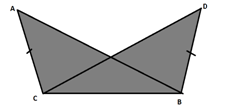Yes, because given that ABC and DBC have side BC common, AB = BD and AC = CD

Also, by SSS standard of congruency, ΔABC ≅ ΔDBC

No, ∠ABD and ∠ACD are not equal because AB not equal to AC

Exercise 16.3

Question :1. By applying SAS congruence condition, state which of the following pairs (Fig. 28) of triangle are congruent. State the result in symbolic form: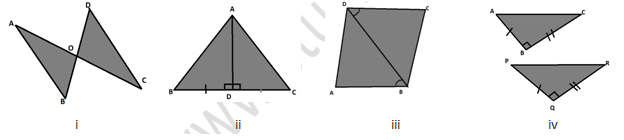Solution 1:

(i) According to the figure OA = OC and OB = OD and

∠AOB = ∠COD which are vertically opposite angles.

Thus. by SAS standard of congruency condition, ΔAOB ≅ΔCOD

(ii) According to the figure we have BD = DC

(iii) According to the AB = DC

∠ABD = ∠CDB and BD = DB

Thus, by SAS standard of congruency condition, ΔABD ≅ΔCBD

(iv) According to the BC = QR

ABC = PQR = 90o

And AB = PQ

Thus, by SAS standard of congruency condition, ΔABC≅ ΔPQR.

Question :2. State the condition by which the following pairs of triangles are congruent.Solution 2:

BC = CD and AC = CA

Thus, by SSS standard of congruency condition, ΔABC≅ ΔADC

(ii) AC = BD

AD = BC and AB = BA

Thus, by SSS standard of congruency condition, ΔABD ≅ ΔBAC

∠BAC = ∠DAC and AC = CA

Thus by SAS standard of congruency condition,  ΔBAC ≅ ΔDAC

∠DAC = ∠BCA and AC = CA

Thus, by SAS standard of congruency condition, ΔABC ≅ ΔADC

Question :3. In fig. 30, line segments AB and CD bisect each other at O. Which of the following statements is true?

(i) ΔAOC ≅ ΔDOB

(ii) ΔAOC ≅ ΔBOD

(iii) ΔAOC ≅ ΔODB

State the three pairs of matching parts, you have used to arrive at the answer.Solution 3:

According to the figure, AO = OB

And, CO = OD

Also, AOC = BOD

Thus, by SAS standard of congruency condition, ΔAOC ≅ ΔBOD

So, the statement (ii) is true.

Question :4. Line-segments AB and CD bisect each other at O. AC and BD are joined forming triangles AOC and BOD. State the three equality relations between the parts of the two triangles that are given or otherwise known. Are the two triangles congruent? State in symbolic form, which congruence condition do you use?

Solution 4:

AO = OB and CO = OD

Then, AB and CD are bisected to each other at point O.

And also ∠AOC = ∠BOD

Then, they are opposite angles on the same vertex.

Thus, by SAS congruence condition, ΔAOC ≅ ΔBOD

Question :5. ΔABC is isosceles with AB = AC. Line segment AD bisects ∠A and meets the base BC in D.

(ii) State the three pairs of matching parts used to answer (i).

(iii) Is it true to say that BD = DC?

Solution 5:

(i)Given in the question AB = AC

Thus, CPCT BD = DC.

Question :6. In Fig. 31, AB = AD and ∠BAC = ∠DAC.

(i) State in symbolic form the congruence of two triangles ABC and ADC that is true.

(ii) Complete each of the following, so as to make it true:

(a) ∠ABC =

(b) ∠ACD =

(c) Line segment AC bisects ….. And ……..Solution 6:

i) In the question given AB = AD;               ∠BAC = ∠DAC; AC = CA (common)

Thus, by SAS standard of congruency condition, ΔABC ≅ ΔADC

∠ACD = ∠ACB (CPCT)

Line segment AC bisects ∠A and ∠C.

Question :7. In fig. 32, AB || DC and AB = DC.

(i) Is ΔACD ≅ ΔCAB?

(ii) State the three pairs of matching parts used to answer (i).

(iii) Which angle is equal to ∠CAD?Solution 7:

(i) Yes, by SAS standard of congruency condition, ΔACD ≅ ΔCAB.

(ii) Three pairs of matching parts: AB = DC, AC = CA and ∠DCA = ∠BAC.

(iii) ∠CAD = ∠ACB then the two triangles are congruent.

(iv) Yes, since the alternative angles are identical, this follows from AD parallel to BC. If the angles of the alternative angles are identical, the lines are parallel.

Exercise 16.4

Question :1. Which of the following pairs of triangles are congruent by ASA condition?Solution 1:

(i) ∠ABO = ∠CDO = 45o and both are alternate angles,

AB parallel to DC, ∠BAO = ∠DCO (alternate angle, AB parallel to CD and AC is a transversal line)

∠ABO = ∠CDO = 45o

AB = DC

As a result, by ASA ΔAOB ≅ ΔDOC

(ii) In ABC,

Now AB =AC

∠ABD = ∠ACD = 40o (∠opposite to equal sides)

∠ABD + ∠ACD + ∠BAC = 180o (∠ sum property)

40o + 40o + ∠BAC = 180o

∠BAC =180o – 80o =100o

∠BAD = ∠BAC – ∠DAC = 100o – 50o = 50o

Thus, by ASA, ΔABD ≅ ΔACD

(iii) In Δ ABC,

∠A + ∠B + ∠C = 180(∠ sum property)

∠C = 180o– ∠A – ∠B

∠C = 180o – 30o – 90o = 60o

In PQR,

∠P + ∠Q + ∠R = 180o (∠ sum property)

∠P = 180o – ∠R – ∠Q

∠P = 180– 60– 90o = 30o

∠BAC = ∠QPR = 30o

∠BCA = ∠PRQ = 60o and AC = PR

As a result, by ASA, ΔABC ≅ ΔPQR

(iv) BC = QR but none of the angles of ΔABC and ΔPQR are equal.

Thus, ΔABC is not congruent to ΔPQR

Question :2. In fig. 37, AD bisects A and AD ⊥ BC.

(ii) State the three pairs of matching parts you have used in (i)

(iii) Is it true to say that BD = DC?Solution 2:

Question :3. Draw any triangle ABC. Use ASA condition to construct another triangle congruent to it.

Solution  3: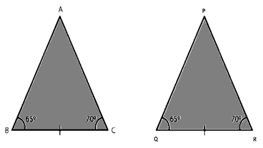Δ ABC with ∠ABC = 65o and ∠ACB = 70o

Now, we construct ΔPQR ≅ ΔABC

where ∠PQR = 65o and ∠PRQ = 70o

and, also construct ΔPQR such that BC = QR

Thus, according to ASA the two triangles are congruent

Question :4. In Δ ABC, it is known that ∠B = C. Imagine you have another copy of Δ ABC

(i) Is ΔABC ≅ ΔACB

(ii) State the three pairs of matching parts you have used to answer (i).

(iii) Is it true to say that AB = AC?

Solution 4: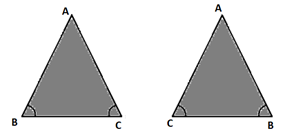(i) Yes, ΔABC ≅ ΔACB

(ii) Three pairs of matching parts: ∠ABC = ∠ACB and ∠ACB = ∠ABC, BC = CB

(iii) Yes, it is true to say that AB = AC since ∠ABC = ∠ACB.

Question :5. In Fig. 38, AX bisects ∠BAC as well as ∠BDC. State the three facts needed to ensure that ΔACD ≅ ΔABDSolution 5:

According to the given conditions,

Thus, According to ASA standard of congruency condition rule, ΔACD ≅ ΔABD

Question :6. In Fig. 39, AO = OB and ∠A = ∠B.

(i) Is ΔAOC ≅ ΔBOD

(ii) State the matching pair you have used, which is not given in the question.

(iii) Is it true to say that ∠ACO = ∠BDO?Solution 6:

∠OAC = ∠OBD,

AO = OB

Also, ∠AOC = ∠BOD         [Opposite angles on same vertex]

Thus, according to ASA standard of congruency condition ΔAOC ≅ ΔBOD

Exercise 16.5

Question :1. In each of the following pairs of right triangles, the measures of some parts are indicated alongside. State by the application of RHS congruence condition which are congruent, and also state each result in symbolic form. (Fig. 46)Solution 1:

(i) ∠ADB = ∠BCA = 90o

Hypotenuse AB = hypotenuse AB

Thus, by RHS ΔADB ≅ ΔACB

Given: Hypotenuse AC = hypotenuse AB

∠ADB = 180o – 90o = 90o

(iii) Hypotenuse AO = hypotenuse DO

BO = CO

∠B = ∠C = 90o

Thus, by RHS, ΔAOB≅ΔDOC

(iv) Hypotenuse AC = Hypotenuse CA

BC = DC

Thus, by RHS, ΔABC ≅ ΔADC

(v) BD = DB

Hypotenuse AB = Hypotenuse BC,

∠BDA + ∠BDC = 180o

∠BDA + 90o = 180o

∠BDA= 180– 90o = 90o

∠BDA = ∠BDC = 90o

Thus, by RHS, ΔABD ≅ ΔCBD

Question :2. Δ ABC is isosceles with AB = AC. AD is the altitude from A on BC.

(i) Is ΔABD ≅ ΔACD?

(ii) State the pairs of matching parts you have used to answer (i).

(iii) Is it true to say that BD = DC?

Solution 2:

(i) Yes, ΔABD ≅ ΔACD by RHS standard of congruency condition

(ii) Hypotenuse AB = Hypotenuse AC

(iii)Yes, it is true to say that BD = DC (CPCT)

Since we have already proved that the two triangles are congruent.

Question :3. ΔABC is isosceles with AB = AC. Also. AD ⊥ BC meeting BC in D. Are the two triangles ABD and ACD congruent? State in symbolic form. Which congruence condition do you use? Which side of ADC equals BD? Which angle of Δ ADC equals ∠B?

Solution 3:

AB = AC - (i)

Common: AD = DA - (ii)

Thus, from (i), (ii) and (iii), by RHS standard of congruency condition, ΔABD ≅ ΔACD, the triangles are congruent.

Thus, BD = CD.

And ∠ABD = ∠ACD (CPCT)

Question :4. Draw a right triangle ABC. Use RHS condition to construct another triangle congruent to it.

Solution  4: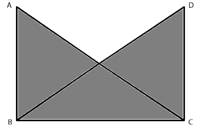Δ ABC with ∠B as right angle.

Construct another triangle on base BC, such that ∠C is a right angle and AB = DC

Also, BC = CB

Thus, by RHS, ΔABC ≅ ΔDCB

Question :5.In fig. 47, BD and CE are altitudes of Δ ABC and BD = CE.

(i) Is ΔBCD ≅ ΔCBE?

(ii) State the three pairs or matching parts you have used to answer (i)Solution 5:

(i) Yes, ΔBCD ≅ ΔCBE by RHS standard of congruency condition.

(ii) Hypotenuse BC = Hypotenuse CB

BD = CE                 [According to the question]

And ∠BDC = ∠CEB = 90o